# JP4567974B2 - Test equipment - Google Patents

## Info

Publication number
JP4567974B2
JP4567974B2 JP2003562654A JP2003562654A JP4567974B2 JP 4567974 B2 JP4567974 B2 JP 4567974B2 JP 2003562654 A JP2003562654 A JP 2003562654A JP 2003562654 A JP2003562654 A JP 2003562654A JP 4567974 B2 JP4567974 B2 JP 4567974B2
Authority
JP
Japan
Prior art keywords
frequency
clock
test
test rate
unit
Prior art date
Legal status (The legal status is an assumption and is not a legal conclusion. Google has not performed a legal analysis and makes no representation as to the accuracy of the status listed.)
Expired - Fee Related
Application number
JP2003562654A
Other languages
Japanese (ja)
Other versions
JPWO2003062843A1 (en
Inventor

Original Assignee

Priority date (The priority date is an assumption and is not a legal conclusion. Google has not performed a legal analysis and makes no representation as to the accuracy of the date listed.)
Filing date
Publication date
Priority to JP2002010877 priority Critical
Priority to JP2002010877 priority
Application filed by 株式会社アドバンテスト filed Critical 株式会社アドバンテスト
Priority to PCT/JP2003/000337 priority patent/WO2003062843A1/en
Publication of JPWO2003062843A1 publication Critical patent/JPWO2003062843A1/en
Application granted granted Critical
Publication of JP4567974B2 publication Critical patent/JP4567974B2/en
Application status is Expired - Fee Related legal-status Critical
Anticipated expiration legal-status Critical

## Images

•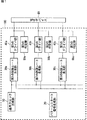•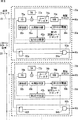•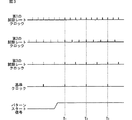•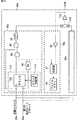•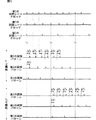•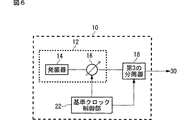•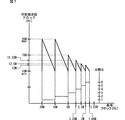••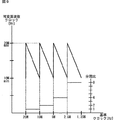•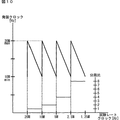•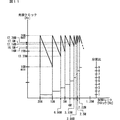## Classifications

• GPHYSICS
• G01MEASURING; TESTING
• G01RMEASURING ELECTRIC VARIABLES; MEASURING MAGNETIC VARIABLES
• G01R31/00Arrangements for testing electric properties; Arrangements for locating electric faults; Arrangements for electrical testing characterised by what is being tested not provided for elsewhere
• G01R31/28Testing of electronic circuits, e.g. by signal tracer
• G01R31/317Testing of digital circuits
• G01R31/3181Functional testing
• G01R31/319Tester hardware, i.e. output processing circuit
• G01R31/31917Stimuli generation or application of test patterns to the device under test [DUT]
• G01R31/31922Timing generation or clock distribution
• GPHYSICS
• G01MEASURING; TESTING
• G01RMEASURING ELECTRIC VARIABLES; MEASURING MAGNETIC VARIABLES
• G01R31/00Arrangements for testing electric properties; Arrangements for locating electric faults; Arrangements for electrical testing characterised by what is being tested not provided for elsewhere
• G01R31/28Testing of electronic circuits, e.g. by signal tracer
• G01R31/317Testing of digital circuits
• G01R31/3181Functional testing
• G01R31/319Tester hardware, i.e. output processing circuit
• G01R31/31917Stimuli generation or application of test patterns to the device under test [DUT]
• G01R31/31928Formatter

## Description

Technical field
The present invention relates to a test apparatus for testing an electronic device. In particular, the present invention relates to a test apparatus for testing an electronic device having a plurality of cores having different operating frequencies. The present application is related to the following Japanese patent application. For designated countries where incorporation by reference of documents is permitted, the contents described in the following application are incorporated into the present application by reference and made a part of the description of the present application.
Japanese Patent Application No. 2002-10877 Application date January 18, 2002
Background art
2. Description of the Related Art Conventionally, a test apparatus for testing an electronic device such as a semiconductor device supplies the electronic device with a test pattern having a frequency corresponding to the operating frequency of the electronic device to test the electronic device. When the electronic device has a plurality of cores having different operating frequencies, the test apparatus sequentially tests each core. For example, when the electronic device has a central processing unit and a decoder having different operating frequencies, the test device supplies test patterns of frequencies corresponding to the respective operating frequencies to the central processing unit and the decoder in order.
However, in order to perform detailed testing of electronic devices, it is necessary to perform testing by operating a plurality of cores simultaneously. Conventionally, a plurality of clocks corresponding to respective operating frequencies of a plurality of cores are generated, and a test pattern corresponding to each core is supplied to each core of the electronic device based on the generated plurality of clocks. . However, in the conventional test apparatus, it is difficult to perform a reproducible test because a plurality of clocks are not synchronized. For example, each time the test is started, the phase relationship of the plurality of test patterns is not reproducible, and it is difficult to perform a reproducible test.
Accordingly, an object of the present invention is to provide a test apparatus that can solve the above-described problems. This object is achieved by a combination of features described in the independent claims. The dependent claims define further advantageous specific examples of the present invention.
Disclosure of the invention
In order to solve the above-described problem, according to a first aspect of the present invention, a test apparatus for testing an electronic device, which is based on a reference clock generating unit that generates a reference clock having a first frequency, and the reference clock And a first test rate generator that generates a first test rate clock having a frequency that is substantially an integer multiple of the first frequency, and a frequency that is substantially an integer multiple of the first frequency based on the reference clock. A second test rate generator for generating a second test rate clock having a frequency different from the frequency of the first test rate clock, and a second frequency according to the first test rate clock. A first driver section for supplying the electronic device with a first test pattern for testing the electronic device, and a third frequency according to the second test rate clock, A second test pattern for testing the scan to provide a test apparatus, characterized in that it comprises a second driver section for supplying the electronic device.
It is preferable that the first driver unit and the second driver unit start supplying the first test pattern and the second test pattern to the electronic device in phase synchronization at a desired timing. In addition, each of the first driver unit and the second driver unit substantially corresponds to the corresponding first test pattern or second test pattern as the frequency of the corresponding first test rate clock or second test rate clock. It may be supplied to the electronic device at the same frequency.
The reference clock generation unit is a least common multiple of a cycle of the first test rate clock to be generated by the first test rate generation unit and a cycle of the second test rate clock to be generated by the second test rate generation unit. Preferably, a reference clock having a period substantially equal to is generated. The test apparatus further includes a pattern start signal generation unit that generates a pattern start signal indicating a desired timing, and the first driver unit and the second driver unit perform the first test based on the pattern start signal. Supply of the pattern and the second test pattern may begin.
Each of the first test rate generation unit and the second test rate generation unit receives a reference clock, generates an oscillation clock having a frequency that is substantially an integer multiple of the frequency of the reference clock, and divides the oscillation clock. The first divider for generating the first test rate clock or the second test rate clock and the first test rate clock or the second test rate clock are divided, and the frequency of the reference clock is abbreviated. A second frequency divider that generates a reference clock having the same frequency, and each oscillation unit has a phase of each oscillation clock based on the phase of each reference clock and the phase of the reference clock. May be synchronized.
Each of the first test rate generation unit and the second test rate generation unit further includes a frequency division control unit that controls a frequency division ratio in the corresponding first frequency divider, and each frequency division control unit includes: The frequency of the first test rate clock or the frequency of the second test rate clock and the frequency of the corresponding oscillation clock to be generated by the corresponding first test rate generation unit or second test rate generation unit Based on this, the frequency division ratio in the corresponding first frequency divider may be controlled. The test apparatus also includes a frequency of the first test rate or the second test rate, a frequency of the oscillation clock, and a frequency to be generated by the first test rate generation unit or the second test rate generation unit. You may further provide the storage part which matches and stores the frequency division ratio in the 1st frequency divider which should be controlled by the circumference control part.
In the test apparatus, each of the first test rate generator and the second test rate generator has a corresponding first test rate clock or second test rate clock based on the reference clock and the pattern start signal. May be further provided with a switching unit that switches whether or not to supply the corresponding first driver unit or second driver unit. When the reference clock and the pattern start signal each indicate a predetermined value, the switching unit sends a corresponding first test rate clock or second signal to the corresponding first driver unit or second driver unit. A test rate clock may be provided.
Each of the first driver unit and the second driver unit delays each pulse in the corresponding first test rate clock or the second test rate clock by a desired integer multiple of the period of the corresponding oscillation clock. A coarse delay unit that generates a timing signal, a pattern generator that generates a first test pattern or a second test pattern corresponding to the timing signal, and a corresponding first test pattern or second test pattern And a fine delay unit that supplies the electronic device with a desired time delay.
The reference clock generation unit generates a variable frequency clock having an octave frequency variable range, and a variable oscillation clock that divides the variable frequency clock by a desired division ratio to generate a reference clock having a desired frequency. A frequency divider.
The above summary of the invention does not enumerate all the necessary features of the present invention, and sub-combinations of these feature groups can also be the invention.
BEST MODE FOR CARRYING OUT THE INVENTION
Hereinafter, the present invention will be described through embodiments of the invention. However, the following embodiments do not limit the invention according to the claims, and all combinations of features described in the embodiments are included. It is not necessarily essential for the solution of the invention.
FIG. 1 shows an example of the configuration of a test apparatus 100 according to the present invention. The test apparatus 100 tests the electronic device 60. The electronic device 60 has a plurality of cores having different operating frequencies. The test apparatus 100 includes a reference clock generation unit 10, a pattern start signal generation unit 20, a plurality of test rate generation units 30, a plurality of driver units 40, and a plurality of determination units 50.
The reference clock generation unit 10 generates a reference clock having a first frequency. The reference clock generator 10 preferably generates a reference clock having a period substantially equal to the least common multiple of the periods of the plurality of test rate clocks to be generated by the respective test rate generators 30.
Of the plurality of test rate generation units 30, the first test rate generation unit 30a generates a first test rate clock having a frequency that is substantially an integer multiple of the first frequency based on the reference clock. Further, the second test rate generation unit 30b has a second test rate having a frequency that is a substantially integer multiple of the first frequency based on the reference clock and that is different from the frequency of the first test rate clock. Generate a clock. Further, the third test rate generation unit 30c has a frequency that is substantially an integer multiple of the first frequency based on the reference clock, and includes the frequency of the first test rate clock and the frequency of the second test rate clock. A third test rate clock having a different frequency is generated.
In the present example, the first test rate generator 30a, the second test rate generator 30b, and the third test rate generator 30c generate test rate clocks having different frequencies, respectively. In the example, one or a plurality of test rate generation units 30 may generate a test rate clock having substantially the same frequency as the frequency of the test rate clock generated by the other test rate generation unit 30. Each test rate generator 30 generates a test rate clock having a frequency corresponding to the operating frequency of the core of the corresponding electronic device.
Among the plurality of driver units 40, the first driver unit 40 a has a second frequency according to the first test rate clock, and applies a first test pattern for testing the electronic device 60 to the electronic device. To supply. In addition, the second driver unit 40 b supplies a second test pattern having a third frequency and testing the electronic device 60 to the electronic device 60 in accordance with the second test rate clock. The third driver unit 40 c supplies the electronic device 60 with a third test pattern for testing the electronic device 60 having a fourth frequency according to the third test rate clock.
Each of the first driver unit 40a, the second driver unit 40b, and the third driver unit 40c corresponds to the corresponding first test pattern, second test pattern, or third test pattern. The first test rate clock, the second test rate clock, or the third test rate clock may be supplied to the electronic device 60 at substantially the same frequency. That is, the plurality of driver units 40 may supply a test pattern having substantially the same frequency as the frequency of the received test rate clock to the corresponding core of the electronic device. For example, the plurality of driver units 40 may supply the pulse included in the test pattern to the core of the corresponding electronic device in accordance with the received pulse of the test rate clock.
The plurality of determination units 50 determine whether the core of the electronic device 60 is good or not based on an output signal output by the core of the corresponding electronic device 60 based on the corresponding test pattern. The plurality of determination units 50 are configured so that the core of the corresponding electronic device 60 outputs the expected value signal to be output based on the corresponding test pattern and the output signal output from the core of the corresponding electronic device 60. It may be judged whether or not. In this case, each of the plurality of driver units 40 may generate an expected value signal to be output by the core of the corresponding electronic device 60 based on the corresponding test pattern and supply the expected value signal to the corresponding determination unit 50.
According to the test apparatus 100 in this example, a plurality of test rate clocks are generated from a single reference clock, and the test pattern is supplied to the electronic device 60 based on the test rate clock. Multiple cores of the electronic device 60 can be tested. For this reason, the test of the electronic device 60 can be accurately performed.
The plurality of driver units 40 preferably start supplying a plurality of test patterns to the electronic device 60 in phase synchronization at a desired timing. In this example, the pattern start signal generation unit 20 generates a pattern start signal indicating a desired timing. The plurality of driver units 40 start supplying a plurality of test patterns based on the pattern start signal. According to the test apparatus 100 in this example, since the supply of a plurality of test patterns is started in phase synchronization at a desired timing, a reproducible test can be performed.
FIG. 2 shows an example of the configuration of the plurality of test rate generation units 30. The plurality of test rate generation units 30 have the same function and configuration. In this example, the configuration of the first test rate generator 30a and the second test rate generator 30b will be described. Each of the first test rate generation unit 30a and the second test rate generation unit 30b includes an oscillation unit 70, a first frequency divider 38, a second frequency divider 42, a switching unit 80, and a frequency division control unit 36. And a storage unit 32.
The oscillation unit 70a receives the reference clock from the reference clock generation unit 10 and generates an oscillation clock having a frequency that is substantially an integer multiple of the frequency of the reference clock. Each oscillation unit 70 synchronizes the phase of each oscillation clock and the test rate clock based on the phase of each reference clock described later and the phase of the reference clock. In this example, each oscillation unit 70 is a phase-locked loop (PLL).
The first frequency divider 38 divides the oscillation clock by a desired frequency division ratio to generate a test rate clock. That is, the first frequency divider 38a of the first test rate generator 30a generates the first test rate clock, and the first frequency divider 38b of the second test rate generator 30b is the second frequency divider. Generate a test rate clock.
Each of the second frequency dividers 42 divides the corresponding test rate clock and generates a reference clock having a frequency substantially the same as the frequency of the reference clock. That is, the second frequency divider 42a of the first test rate generation unit 30a divides the first test rate clock, generates a reference clock having a frequency substantially the same as the frequency of the reference clock, and the second The second frequency divider 42b of the test rate generator 30b divides the second test rate clock and generates a reference clock having a frequency substantially the same as the frequency of the reference clock.
The oscillation unit 70 includes a phase detector 72, a loop filter 74, a DA 77, and a voltage controlled oscillator 76. The voltage controlled oscillator 76 generates an oscillation clock having a desired frequency. The phase detector 72 detects the phase of the reference clock and the phase of the reference clock. Based on the difference between the phase of the reference clock detected by the phase detector 72 and the phase of the reference clock, the loop filter 74 controls the oscillation frequency of the oscillation clock generated by the voltage controlled oscillator 76 so that the difference decreases. .
Further, the DA 77 gives an offset for correcting the skew of the test rate clock due to component variation or the like. For example, the DA 77a and the DA 77b respectively delay the signal output from the phase detector 72 by a predetermined time, and correct the skew between the first test rate clock and the second test rate clock.
The oscillator 70 synchronizes the phase of the reference clock with the phase of the oscillation clock and the phase of the test rate clock by synchronizing the phase of the reference clock with the phase of the reference clock. Since the reference clock and the reference clock have substantially the same frequency, the phase of the reference clock and the phase of the reference clock can be accurately synchronized. Therefore, the oscillation unit 70 can generate an oscillation clock and a test rate clock that are accurately synchronized with the reference clock. Further, since each oscillating unit 70 synchronizes the same reference clock and each test rate clock, the test rate clock generated by each oscillating unit 70 can be synchronized. That is, the plurality of oscillation units 70 can generate a plurality of test rate clocks having different frequencies and having phases synchronized at a predetermined timing. In this example, the loop filter 74 is used to synchronize the reference clock and the respective test rate clocks. In other examples, the reference clock and the respective test rate clocks are synchronized using other methods. May be synchronized.
Further, the frequency division control unit 36 controls the frequency division ratio in the corresponding first frequency divider 38 and the frequency division ratio in the corresponding second frequency divider 42. Each frequency division control unit 36 divides the frequency in the corresponding first frequency divider 38 based on the frequency of the test rate clock and the frequency of the corresponding oscillation clock that the corresponding test rate generation unit 30 should generate. The frequency ratio and the frequency division ratio in the corresponding second frequency divider 42 are controlled.
The frequency division control unit 36 controls the frequency division ratio in the first frequency divider 38, so that a test rate clock having a desired frequency can be generated from an oscillation clock having a desired frequency. Further, the frequency division control unit 36 controls the frequency division ratio in the second frequency divider 42 to generate a reference clock having a frequency substantially equal to the frequency of the reference clock and an oscillation clock having a desired frequency. Can do. For example, when it is desired to reduce the jitter in the oscillation unit 70, the oscillation unit 70 may lower the frequency of the oscillation clock. When it is desired to reduce the variable error in the fine delay unit 92 described later, the oscillation unit 70 may increase the frequency of the oscillation clock. The frequency division control unit 36 controls the frequency division ratio in the first frequency divider 38 and the second frequency divider 42 based on the frequency of the oscillation clock. The test apparatus 100 further includes an instruction unit that receives an instruction from a user to reduce the jitter in the oscillation unit 70 or to reduce the variable error in the fine delay unit 92. The frequency division control unit 36 is based on the instruction. , Respectively, may control the first frequency divider 38 and / or the second frequency divider 42.
In addition, the storage unit 32 includes a first frequency divider to be controlled by the frequency of the test rate to be generated by the corresponding test rate generation unit 30, a frequency of the corresponding oscillation clock, and the corresponding frequency division control unit 36. A table in which the frequency division ratio at 38 and the frequency division ratio at the second frequency divider 42 are associated with each other is stored. For example, the test apparatus 100 may further include an instruction unit that receives information on the frequency of the test rate to be generated by each test rate generation unit 30 and information on the frequency of the oscillation clock from the outside. The frequency division control unit 36 refers to the table stored in the storage unit 32 based on the information received by the instruction unit, and controls the frequency division ratio in the first frequency divider 38 and the second frequency divider 42. . Further, the frequency of the oscillation clock may be determined in advance.
Whether each switching unit 80 supplies the test rate clock generated by the corresponding test rate generating unit 30 to the corresponding driver unit 40 (see FIG. 1) based on the reference clock and the pattern start signal. Switch. The switching unit 80 supplies a corresponding test rate clock to the corresponding driver unit 40 when each of the reference clock and the pattern start signal indicates a predetermined value. In this example, the switching unit 80 includes a flip-flop 82 and an AND circuit 84. The flip-flop 82 is a D-FF that receives the reference clock and the pattern start signal and outputs the pattern start signal when the reference clock indicates a desired value. The AND circuit 84 supplies the corresponding test rate clock to the corresponding driver unit 40 when the output of the flip-flop 82 indicates H logic.
Each switching unit 80 switches whether or not to supply the corresponding test rate clock to the corresponding driver unit 40 based on the pattern start signal and the reference clock, so that each driver unit 40 controls the electronic device 60. Thus, the timing for starting the supply of the test pattern can be synchronized. Further, in this example, the configurations of the first test rate generation unit 30a and the second test rate generation unit 30b have been described, but the other test rate generation units 30 also have the same functions and configurations. That is, according to the test apparatus 100 in this example, a plurality of test rate clocks having different phases and different frequencies can be generated. Therefore, a plurality of test patterns having different frequencies can be supplied to the electronic device 60 in phase synchronization.
FIG. 3 is a timing chart illustrating an example of a plurality of test rate clocks generated by the plurality of test rate generation units 30. In FIG. 3, the horizontal axis indicates the time axis. The reference clock generation unit 10 (see FIG. 1) generates a reference clock having a period substantially equal to the least common multiple of the periods of the plurality of test rate clocks to be generated by each test rate generation unit 30. Here, the period equal to the least common multiple refers to the minimum period divisible by the period of each test rate, and the period of the reference clock and the test rate clock may be represented by a decimal. In this example, the frequency of the reference clock is 1 MHz, the frequency of the first test rate clock is 4 MHz, the frequency of the second test rate clock is 3 MHz, and the frequency of the third test rate clock is 2 MHz.
As described in FIG. 2, the first test rate clock, the second test rate clock, and the third test rate clock are synchronized with the reference clock as shown in FIG. In this example, the plurality of test rate clocks are pulse timings of the reference clock (T 1 , T 2 , T 3 However, in another example, the plurality of test rate clocks may be synchronized at a timing delayed by a desired offset time from the timing of the reference clock pulse. For example, the test rate generation unit 30 described with reference to FIG. 2 may each include a delay circuit that delays the test rate by a desired offset time.
The plurality of test rate generators 30 have a timing (T) in which the pattern start signal indicates H logic and the reference clock indicates H logic. 1 ) Starts supplying the test rate clock to the corresponding driver unit 40 (see FIG. 1). The driver unit 40 supplies a test pattern according to the pulse of the test rate clock. In this example, the driver unit 40 shapes a signal obtained by delaying a pulse of the test rate clock for a desired time, and supplies the signal to the electronic device 60 as a test pattern.
Further, the pattern start signal generation unit 20 starts the pattern start where the value changes to H logic based on the timing when one cycle of the test pattern starts and the value changes to L logic based on the timing when the test pattern ends. Generate a signal. Since the reference clock generation unit 10 generates a reference clock having a period substantially the same as the least common multiple of the periods of the plurality of test rate clocks, even if the test pattern cycle is performed continuously, The cycle can be started efficiently. That is, the supply of the test pattern of the next cycle can be started at the timing when the plurality of test rate clocks are synchronized immediately after the end of the current cycle.
FIG. 4 shows an example of the configuration of the driver unit 40. FIG. 4 illustrates the configuration of the first driver unit 40a. The other driver unit 40 has the same or similar function and configuration as the first driver unit 40a. The plurality of drive units 40 include a set side generation unit 90a, a reset side generation unit 90b, a set reset latch 116, a determination unit 50, and a driver 118.
The set-side generation unit 90a generates the rising edge timing of the test pattern waveform, and the reset-side generation unit 90b generates the falling edge timing of the test pattern waveform. The set-side generation unit 90a and the reset-side generation unit 90b have the same or similar functions and configurations.
The set reset latch 116 generates a test pattern whose value changes to H logic at the timing generated by the set side generation unit 90a and whose value changes to L logic at the timing generated by the reset side generation unit 90b. The driver 118 supplies the test pattern generated by the set / reset latch 116 to the electronic device 60.
The set side generation unit 90a includes a coarse delay unit 110, a pattern generator 94, an AND circuit (96, 98), and a fine delay unit 92. The coarse delay unit 110 generates a timing signal obtained by delaying each pulse in the corresponding test rate clock by a desired integer multiple of the period of the corresponding oscillation clock. The pattern generator 94 generates a pulse indicating the rising edge of the corresponding test pattern in accordance with the test rate clock. The AND circuit 96 and the AND circuit 98 shape the pulse into a burst signal. The fine delay unit 92 delays a pulse indicating the rising edge of the test pattern by a desired time and supplies the pulse to the set / reset latch 116.
The coarse delay unit 110 includes a counter 112 and a counter control unit 114. The counter 112 uses the test rate clock as a trigger, counts the number of pulses of the oscillation clock, and outputs a predetermined pulse when the desired number of pulses is counted. The counter control unit 114 controls the number of pulses that the counter 112 should count. By controlling the number of pulses that the counter 112 should count by the counter control unit 114, it is possible to generate a pulse by delaying the pulse of the test rate clock by a desired integer multiple of the period of the oscillation clock.
The fine delay unit 92 delays the pulse generated by the AND circuit 98 for a desired time smaller than the cycle of the oscillation clock. The fine delay unit 92 includes a variable delay circuit and a linearized memory that stores a table for controlling a delay amount in the variable delay circuit. The fine delay unit 92 generates a delay with a desired time smaller than the oscillation clock cycle, and therefore the capacity of the linearized memory can be reduced by setting the frequency of the oscillation clock high in advance. According to this example, a test pattern having a desired phase can be generated.
The determination unit 50 determines pass / fail of the electronic device 60 based on an output signal output by the electronic device 60 according to the test pattern. The determination unit 50 includes a comparator 56, a comparator 52, and a fail memory 54.
The comparator 56 supplies a signal indicating whether or not the output signal is greater than a predetermined threshold value to the comparator 52 as a digital signal. The comparator 52 compares the digital signal received from the comparator 56 with the expected value signal received from the pattern generator 94, and determines the quality of the corresponding core of the electronic device 60. The fail memory 54 stores the determination result in the comparator 52.
FIG. 5 shows a timing chart of an example of a plurality of test patterns generated by the plurality of driver units 40. In FIG. 5, the horizontal axis indicates the time axis. Further, the test rate clock shown in FIG. 5 has the same phase as the test rate clock shown in FIG.
Each driver unit 40 generates a test pattern having a pulse delayed by a desired time from each pulse of the corresponding test rate clock. For example, as shown in FIG. 5, the first driver unit 40 a starts ΔT from the pulse of the first test rate clock. 1 , ΔT 2 A test pattern having a pulse delayed by a time is generated. Since the plurality of test rate clocks are synchronized at a predetermined timing, the plurality of test patterns are also synchronized at a predetermined timing.
In addition, T shown in FIG. 1 , T 2 By starting the test cycle at the timing when the plurality of test rate clocks such as... Are synchronized, a plurality of test patterns in each test cycle can be supplied to the electronic device 60 in synchronization. . For example, in each driver unit 40, T 1 The test pattern of the first cycle is started at the timing shown in FIG. 2 By starting the test pattern of the first cycle at the timing shown in (frequency of test rate clock [Hz] / frequency of reference clock [Hz]) + 1 cycle, a plurality of test patterns in each test cycle can be synchronized. The test apparatus 100 controls the timing of starting each test cycle by the pattern start signal generated by the pattern start signal generation unit 20 (see FIG. 1).
T 1 The phase relationship between a plurality of test patterns when the test pattern supply is started at the timing 2 As shown in FIG. 5, the phase relationship with a plurality of test patterns when the supply of the test pattern is started at the timing is substantially the same. For this reason, even when the supply of the test pattern is started at an arbitrary timing, a reproducible test can be performed.
FIG. 6 shows an example of the configuration of the reference clock generation unit 10. The reference clock generation unit 10 includes a variable oscillation unit 12, a third frequency divider 18, and a reference clock control unit 22.
The variable oscillation unit 12 generates a variable frequency clock having an octave frequency variable range. For example, the variable oscillation unit 12 generates a variable frequency clock having a frequency variable range of 10 MHz to 20 MHz.
The third frequency divider 18 divides the variable frequency clock by a desired frequency division ratio to generate a reference clock having a desired frequency. The third frequency divider 18 generates a reference clock having a period of the least common multiple of the periods of the plurality of test rate clocks described above.
For example, the third frequency divider 18 can divide by a division ratio of 1, 2,..., 6, and the variable oscillation unit 12 generates a variable frequency clock having a frequency variable range of 10 MHz to 20 MHz. In this case, the reference clock generation unit 10 can generate a reference clock having an arbitrary frequency between 1.66 MHz and 20 MHz. The reference clock control unit 22 controls the oscillation frequency in the variable oscillation unit 12 and the frequency division ratio in the third frequency divider 18 based on the frequency of the reference clock to be generated.
The variable oscillating unit 12 includes an oscillator 14 and an octave variable device 16. The oscillator 14 generates a clock having a predetermined frequency. The octave variable unit 16 generates a variable frequency clock having an octave frequency based on the clock generated by the oscillator 14.
FIG. 7 illustrates an example of a control method in the reference clock control unit 22. In FIG. 7, the left vertical axis indicates the frequency of the variable frequency clock, the horizontal axis indicates the frequency of the reference clock, and the right vertical axis indicates the frequency division ratio in the third frequency divider 18. In this example, the variable frequency clock has a variable frequency region of 10 MHz to 20 MHz, and the third frequency divider 18 can set a frequency dividing ratio of 1, 2, 3,.
The reference clock control unit 22 first sets the frequency division ratio in the third frequency divider 18 based on the frequency of the reference clock to be generated. For example, when it is desired to generate an 8 MHz reference clock, the reference clock control unit 22 sets the frequency division ratio in the third frequency divider 18 to 2 as shown in FIG. The reference clock control unit 22 may set the frequency division ratio in the third frequency divider 18 based on the following mathematical formula.
Where M is the frequency division ratio in the third frequency divider 18, and f0 min Is the lower limit of the variable frequency clock (10 MHz in this example), f ref Indicates the frequency of the reference clock to be generated.
Next, the reference clock control unit 22 controls the frequency of the variable frequency clock generated by the variable oscillation unit 12 based on the set frequency division ratio and the frequency of the reference clock. For example, when it is desired to generate an 8 MHz reference clock, the reference clock control unit 22 controls the frequency of the variable frequency clock generated by the variable oscillation unit 12 to 16 MHz as shown in FIG. The reference clock control unit 22 may control the frequency of the variable frequency clock generated by the variable oscillation unit 12 based on the following mathematical formula.
However, f0 shows the frequency of a variable frequency clock.
FIG. 8 illustrates an example of a control method of the frequency division control unit 36. In FIG. 8, the left vertical axis indicates the frequency of the oscillation clock, the horizontal axis indicates the frequency of the test rate clock, and the right vertical axis indicates the frequency division ratio in the first frequency divider 38. In this example, the oscillation clock has a variable frequency region of 10 MHz to 20 MHz, and the first frequency divider 38 can be set with a frequency division ratio of 1, 2, 3,.
The frequency division control unit 36 sets the frequency division ratio in the first frequency divider 38 based on the frequency of the test rate clock to be generated. For example, when it is desired to generate a 3 MHz test rate clock, the frequency division control unit 36 sets the frequency division ratio in the first frequency divider 38 to 4 as shown in FIG. The frequency division control unit 36 may set the frequency division ratio in the first frequency divider 38 based on the following mathematical formula.
However, La is the frequency division ratio in the first frequency divider 38, f1 min Is the lower limit of the oscillation clock (10 MHz in this example), f rate Indicates the frequency of the test rate clock to be generated.
The oscillation unit 70 generates an oscillation clock having a frequency based on the frequency division ratio set by the frequency division control unit 36 and the frequency of the test rate clock. For example, when it is desired to generate a 3 MHz test rate clock, the oscillation unit 70 generates an oscillation clock having a frequency of 12 MHz as shown in FIG. The oscillation unit 70 may generate an oscillation clock having a frequency based on the following mathematical formula.
Here, f1 indicates the frequency of the oscillation clock.
FIG. 9 illustrates another example of the control method in the reference clock control unit 22. In FIG. 9, the left vertical axis indicates the frequency of the variable frequency clock, the horizontal axis indicates the frequency of the reference clock, and the right vertical axis indicates the frequency division ratio in the third frequency divider 18. In this example, the variable frequency clock has a variable frequency region of 10 MHz to 20 MHz, and the third frequency divider 18 includes 1, 2, 4,..., 8,. m The division ratio can be set.
Also in this example, the reference clock control unit 22 performs the same control as the reference clock control unit 22 described in FIG. In this example, the reference clock control unit 22 sets the frequency division ratio in the third frequency divider 18 based on the following mathematical formula.
Where M is the frequency division ratio in the third frequency divider 18, and f0 min Is the lower limit of the variable frequency clock (10 MHz in this example), f ref Indicates the frequency of the reference clock to be generated.
Further, the reference clock control unit 22 is configured so that the variable oscillation unit 12 is based on the following mathematical formula.
However, f0 shows the frequency of a variable frequency clock. According to this example, simplification of the circuit can be realized.
FIG. 10 illustrates another example of the control method in the frequency division control unit 36. In FIG. 10, the left vertical axis indicates the frequency of the oscillation clock, the horizontal axis indicates the frequency of the test rate clock, and the right vertical axis indicates the frequency division ratio in the first frequency divider 38. In this example, the oscillation clock has a variable frequency region of 10 MHz to 20 MHz, and the first frequency divider 38 can be set with a frequency division ratio of 1, 2, 3,.
Also in this example, the frequency division control unit 36 performs the same control as the frequency division control unit 36 described in FIG. In this example, the frequency division control unit 36 sets the frequency division ratio La in the first frequency divider 38 based on the following mathematical formula.
However, L is represented by the following formula.
However, f1 min Is the lower limit of the oscillation clock (10 MHz in this example), f rate Indicates the frequency of the test rate clock to be generated.
Also in this example, the oscillation unit 70 performs the same control as the oscillation unit 70 described in FIG. In this example, the oscillation unit 70 generates an oscillation clock having a frequency based on the following mathematical formula.
Here, f1 indicates the frequency of the oscillation clock. According to this example, simplification of the circuit can be realized.
FIG. 11 illustrates still another example of the control method in the frequency division control unit 36. In FIG. 11, the left vertical axis indicates the frequency of the oscillation clock, the horizontal axis indicates the frequency of the test rate clock, and the right vertical axis indicates the frequency division ratio in the first frequency divider 38. In this example, the oscillation clock has a variable frequency region of 10 MHz to 20 MHz, and the first frequency divider 38 can be set with a frequency division ratio of 1, 2, 3,.
Also in this example, the frequency division control unit 36 performs the same control as the frequency division control unit 36 described in FIG. In this example, the frequency division control unit 36 sets the frequency division ratio in the first frequency divider 38 based on the following mathematical formula.
However, La is the frequency division ratio in the first frequency divider 38, f1 max Is the upper limit of the oscillation clock (20 MHz in this example), f rate Indicates the frequency of the test rate clock to be generated.
Also in this example, the oscillation unit 70 performs the same control as the oscillation unit 70 described in FIG. In this example, the oscillation unit 70 generates an oscillation clock having a frequency based on the following mathematical formula.
Here, f1 indicates the frequency of the oscillation clock.
In the example described with reference to FIG. 8, since the frequency division ratio in the first frequency divider 38 is set low and the frequency of the oscillation clock is set low, the jitter in the oscillation unit 70 can be reduced as described above. . In the example described with reference to FIG. 11, since the frequency dividing ratio in the first frequency divider 38 is set high and the frequency of the oscillation clock is set high, the variable delay error in the fine delay unit 92 is reduced as described above. can do.
As mentioned above, although this invention was demonstrated using embodiment, the technical scope of this invention is not limited to the range as described in the said embodiment. Various modifications or improvements can be added to the above embodiment. It is apparent from the scope of the claims that the embodiments added with such changes or improvements can be included in the technical scope of the present invention.
As mentioned above, although this invention was demonstrated using embodiment, the technical scope of this invention is not limited to the range as described in the said embodiment. It will be apparent to those skilled in the art that various modifications or improvements can be added to the above embodiment. It is apparent from the scope of the claims that the embodiments added with such changes or improvements can be included in the technical scope of the present invention.
Industrial applicability
As is apparent from the above description, according to the present invention, an electronic device having a plurality of cores having different operating frequencies can be tested with high accuracy.
[Brief description of the drawings]
FIG. 1 is a diagram showing an example of the configuration of a test apparatus 100 according to the present invention.
FIG. 2 is a diagram illustrating an example of the configuration of the plurality of test rate generation units 30.
FIG. 3 is a diagram illustrating a timing chart of an example of a plurality of test rate clocks generated by the plurality of test rate generation units 30.
FIG. 4 is a diagram illustrating an example of the configuration of the driver unit 40.
FIG. 5 is a diagram illustrating a timing chart of an example of a plurality of test patterns generated by the plurality of driver units 40.
FIG. 6 is a diagram illustrating an example of the configuration of the reference clock generation unit 10.
FIG. 7 is a diagram for explaining an example of a control method in the reference clock control unit 22.
FIG. 8 is a diagram for explaining an example of a control method of the frequency division control unit 36.
FIG. 9 is a diagram for explaining another example of the control method in the reference clock control unit 22.
FIG. 10 is a diagram for explaining another example of the control method in the frequency division control unit 36.
FIG. 11 is a diagram illustrating still another example of the control method in the frequency division control unit 36.

## Claims (5)

1. A test apparatus for testing an electronic device having a plurality of cores having different operating frequencies,
A reference clock generator for generating a reference clock having a first frequency;
A first test rate generator that generates a first test rate clock having a frequency that is substantially an integer multiple of the first frequency based on the reference clock;
Based on the reference clock, a second test rate that generates a second test rate clock having a frequency that is a substantially integer multiple of the first frequency and that is different from the frequency of the first test rate clock. A generator,
A first driver section having a second frequency in response to the first test rate clock and supplying a first test pattern for testing the electronic device to the first core of the electronic device; ,
A second driver section having a third frequency in response to the second test rate clock and supplying a second test pattern for testing the electronic device to a second core of the electronic device; ,
Based on said first signal core output of the electronic device, and the determining the quality of the first core 1 of the determination unit,
A second determination unit that determines the quality of the second core based on a signal output by the second core of the electronic device ;
A pattern start signal generating unit for generating a pattern start signal indicating a predetermined timing , and
The first driver unit and the second driver unit phase-synchronize the supply of the first test pattern and the second test pattern to the electronic device at the predetermined timing based on the pattern start signal. Start,
Each of the first test rate generator and the second test rate generator is
An oscillation unit that receives the reference clock and generates an oscillation clock having a frequency that is substantially an integer multiple of the frequency of the reference clock;
A first divider for dividing the oscillation clock to generate the first test rate clock or the second test rate clock;
A second frequency divider that divides the first test rate clock or the second test rate clock and generates a reference clock having a frequency substantially the same as the frequency of the reference clock;
Based on the reference clock and the pattern start signal, the corresponding first test rate clock or the second test rate clock is supplied to the corresponding first driver unit or the second driver unit. A switching unit for switching whether or not to
Have
Each of the oscillation units synchronizes the phase of each oscillation clock based on the phase of each reference clock and the phase of the reference clock .
2. Each of the first test rate generation unit and the second test rate generation unit further includes a frequency division control unit that controls a frequency division ratio in the corresponding first frequency divider,
Each of the frequency division control units has the frequency of the first test rate clock or the second test rate clock to be generated by the corresponding first test rate generation unit or second test rate generation unit. 2. The test apparatus according to claim 1 , wherein a frequency division ratio in the corresponding first frequency divider is controlled based on the frequency of the first frequency divider and the frequency of the corresponding oscillation clock.
3. The frequency of the first test rate or the frequency of the second test rate to be generated by the first test rate generator or the second test rate generator;
The frequency of the oscillation clock;
The test apparatus according to claim 2 , further comprising a storage unit that associates and stores a frequency division ratio in the first frequency divider to be controlled by the frequency division control unit.
4. Each of the first driver unit and the second driver unit is
A coarse delay unit for generating a timing signal obtained by delaying each pulse in the corresponding first test rate clock or the second test rate clock by a predetermined integer multiple of the period of the corresponding oscillation clock;
A pattern generator for generating the corresponding first test pattern or the second test pattern in response to the timing signal;
The test apparatus according to claim 1 , further comprising a fine delay unit that delays the corresponding first test pattern or the second test pattern by a predetermined time and supplies the delayed pattern to the electronic device.
5. The reference clock generation unit
A variable oscillation unit for generating a variable frequency clock having a frequency variable range of an octave;
The test apparatus according to claim 4 , further comprising: a third frequency divider that divides the variable frequency clock by a predetermined frequency division ratio and generates the reference clock having a predetermined frequency.
JP2003562654A 2002-01-18 2003-01-17 Test equipment Expired - Fee Related JP4567974B2 (en)

## Priority Applications (3)

Application Number Priority Date Filing Date Title
JP2002010877 2002-01-18
JP2002010877 2002-01-18
PCT/JP2003/000337 WO2003062843A1 (en) 2002-01-18 2003-01-17 Tester

## Publications (2)

Publication Number Publication Date
JPWO2003062843A1 JPWO2003062843A1 (en) 2005-05-26
JP4567974B2 true JP4567974B2 (en) 2010-10-27

# Family

## Family Applications (1)

Application Number Title Priority Date Filing Date
JP2003562654A Expired - Fee Related JP4567974B2 (en) 2002-01-18 2003-01-17 Test equipment

## Country Status (4)

US (1) US6956395B2 (en)
JP (1) JP4567974B2 (en)
DE (1) DE10392225T5 (en)
WO (1) WO2003062843A1 (en)

## Families Citing this family (29)

* Cited by examiner, † Cited by third party
Publication number Priority date Publication date Assignee Title
JP4729251B2 (en) * 2003-11-28 2011-07-20 株式会社アドバンテスト High frequency delay circuit and test apparatus
JP4477388B2 (en) * 2004-03-24 2010-06-09 Ｎｅｃエレクトロニクス株式会社 Integrated circuit device and evaluation method thereof
EP1610137B1 (en) * 2004-06-24 2009-05-20 Verigy (Singapore) Pte. Ltd. Per-pin clock synthesis
JP2006038743A (en) * 2004-07-29 2006-02-09 Nec Electronics Corp Semiconductor integrated circuit device and testing device thereof
US7319936B2 (en) * 2004-11-22 2008-01-15 Teradyne, Inc. Instrument with interface for synchronization in automatic test equipment
KR100657830B1 (en) * 2005-01-24 2006-12-14 삼성전자주식회사 Apparatus and Method for test Semiconductor Memory Device
US7587645B2 (en) * 2005-01-24 2009-09-08 Samsung Electronics Co., Ltd. Input circuit of semiconductor memory device and test system having the same
US7202656B1 (en) * 2005-02-18 2007-04-10 Lsi Logic Corporation Methods and structure for improved high-speed TDF testing using on-chip PLL
JP4621050B2 (en) * 2005-03-28 2011-01-26 株式会社アドバンテスト Clock transfer device and test device
US7940921B2 (en) * 2005-06-23 2011-05-10 Agere Systems Inc. Continuous power transfer scheme for two-wire serial link
US7773733B2 (en) * 2005-06-23 2010-08-10 Agere Systems Inc. Single-transformer digital isolation barrier
WO2007001930A2 (en) * 2005-06-23 2007-01-04 Agere Systems Inc. Serial protocol for agile sample rate switching
US8213489B2 (en) 2005-06-23 2012-07-03 Agere Systems Inc. Serial protocol for agile sample rate switching
US7366939B2 (en) * 2005-08-03 2008-04-29 Advantest Corporation Providing precise timing control between multiple standardized test instrumentation chassis
US7437588B2 (en) * 2005-08-03 2008-10-14 Advantest Corporation Circuit card synchronization within a standardized test instrumentation chassis
US7437589B2 (en) * 2005-08-03 2008-10-14 Advantest Corporation Providing precise timing control within a standardized test instrumentation chassis
US7257508B2 (en) * 2005-09-09 2007-08-14 Advantest Corporation Timing generator, and timing generating method
JP4806599B2 (en) * 2006-07-20 2011-11-02 株式会社アドバンテスト Electrical circuits and test equipment
DE112007003424T5 (en) 2007-03-27 2010-01-21 Advantest Corp. tester
US7797121B2 (en) * 2007-06-07 2010-09-14 Advantest Corporation Test apparatus, and device for calibration
CN102124357A (en) * 2008-08-19 2011-07-13 爱德万测试株式会社 Test device and testing method
JP5134089B2 (en) * 2008-09-17 2013-01-30 株式会社アドバンテスト Test apparatus and inter-domain synchronization method
US8692566B2 (en) 2008-12-08 2014-04-08 Advantest Corporation Test apparatus and test method
US8261119B2 (en) 2009-09-10 2012-09-04 Advantest Corporation Test apparatus for testing device has synchronization module which synchronizes analog test module to digital test module based on synchronization signal received from digital test module
US8405415B2 (en) 2009-09-10 2013-03-26 Advantest Corporation Test apparatus synchronous module and synchronous method
US7906981B1 (en) 2009-09-10 2011-03-15 Advantest Corporation Test apparatus and test method
US20110184687A1 (en) * 2010-01-25 2011-07-28 Advantest Corporation Test apparatus and test method
JP6268020B2 (en) * 2014-03-26 2018-01-24 ラピスセミコンダクタ株式会社 Clock generation method and semiconductor device
JP2017174109A (en) * 2016-03-23 2017-09-28 株式会社メガチップス Signal generator and variation suppression method of output voltage of regulator

## Citations (3)

* Cited by examiner, † Cited by third party
Publication number Priority date Publication date Assignee Title
JPS61142476A (en) * 1984-12-17 1986-06-30 Hitachi Ltd Pattern generator
JPH10300827A (en) * 1997-04-21 1998-11-13 Ando Electric Co Ltd Ic tester and ic testing method
JPH1114714A (en) * 1997-06-24 1999-01-22 Advantest Corp Semiconductor test equipment

## Family Cites Families (12)

* Cited by examiner, † Cited by third party
Publication number Priority date Publication date Assignee Title
FR2632639B1 (en) 1988-06-09 1990-10-05 Sanofi Sa Derivatives of amino-4-carboxy-3 naphthyridines, their preparation and pharmaceutical compositions containing them
JPH082624Y2 (en) * 1988-08-24 1996-01-29 日本電気株式会社 Test equipment
JPH03195978A (en) * 1989-12-25 1991-08-27 Fujitsu Ltd Semiconductor device equipped with internal clock forming circuit
JPH0862308A (en) * 1994-08-22 1996-03-08 Advantest Corp Timing calibration method for measuring signal of semiconductor testing device and its circuit
JP3574696B2 (en) * 1995-05-26 2004-10-06 株式会社アドバンテスト Timing generator for IC tester
JPH0926467A (en) * 1995-07-11 1997-01-28 Advantest Corp Pll oscillator for timing generation circuit of ic tester
US6404220B1 (en) * 1997-11-20 2002-06-11 Advantest Corporation IC testing method and IC testing device using the same
JP3392029B2 (en) * 1997-12-12 2003-03-31 株式会社アドバンテスト IC tester voltage applied current measuring circuit
JP4386514B2 (en) * 1998-11-24 2009-12-16 株式会社アドバンテスト Semiconductor test equipment
JP2001201533A (en) * 2000-01-21 2001-07-27 Mitsubishi Electric Corp Semiconductor device with built-in burn-in circuit and test method thereof
JP2001244923A (en) * 2000-02-29 2001-09-07 Toyo Commun Equip Co Ltd Clock generating circuit
JP4771572B2 (en) * 2000-04-10 2011-09-14 富士通セミコンダクター株式会社 PLL semiconductor device and method and apparatus for testing the same

## Patent Citations (3)

* Cited by examiner, † Cited by third party
Publication number Priority date Publication date Assignee Title
JPS61142476A (en) * 1984-12-17 1986-06-30 Hitachi Ltd Pattern generator
JPH10300827A (en) * 1997-04-21 1998-11-13 Ando Electric Co Ltd Ic tester and ic testing method
JPH1114714A (en) * 1997-06-24 1999-01-22 Advantest Corp Semiconductor test equipment

## Also Published As

Publication number Publication date
DE10392225T5 (en) 2005-01-27
US6956395B2 (en) 2005-10-18
US20040239310A1 (en) 2004-12-02
WO2003062843A1 (en) 2003-07-31
JPWO2003062843A1 (en) 2005-05-26

## Similar Documents

Publication Publication Date Title
EP1160984B1 (en) Phase-locked loop with improved trade-off between lock-up time and power dissipation
DE69828300T2 (en) Digital frequency synthesis through sequential approaches of fraction parts
US6580304B1 (en) Apparatus and method for introducing signal delay
JP3587818B2 (en) Phase control circuit
DE60020982T2 (en) Device for automatic compensation of a spreading spectrum actuator and method therefor
KR100411551B1 (en) Wide frequency-range delay-locked loop circuit
CN1175571C (en) Delay circuit, clock generating circuit and phase synchronized circuit
EP0727877B1 (en) Fast frequency switching synthesizer
KR100232271B1 (en) Auto-lock circuit for pll frequency synthesizers
US20040257124A1 (en) Spread spectrum clock generator capable of frequency modulation with high accuracy
TW525348B (en) Digital PLL circuit and clock generation method
EP0453280B1 (en) PLL frequency synthesizer
US5781054A (en) Digital phase correcting apparatus
KR100201709B1 (en) Timing signal generating circuit
JP4649362B2 (en) Oscillator control device
US7093177B2 (en) Low-jitter clock for test system
US5095262A (en) Electro-optic sampling system clock and stimulus pattern generator
CN1126318C (en) Clock delay circuitry and oscillation circuitry and phase synchronization circuitry using clock delay circuitry
US5959479A (en) Sampling timebase system
EP0218406A2 (en) Sampling clock generation circuit
US6157694A (en) Fractional frequency divider
JP3406439B2 (en) Variable delay circuit delay time measurement device
US6597753B1 (en) Delay clock generating apparatus and delay time measuring apparatus
JP2004509421A (en) Segmented spectrum clock generator device and method of using same
JP2002518869A (en) Method and apparatus for adjusting the bandwidth of the phase locked loop

## Legal Events

Date Code Title Description
A621 Written request for application examination

Free format text: JAPANESE INTERMEDIATE CODE: A621

Effective date: 20050927

A131 Notification of reasons for refusal

Free format text: JAPANESE INTERMEDIATE CODE: A131

Effective date: 20090224

A521 Written amendment

Free format text: JAPANESE INTERMEDIATE CODE: A523

Effective date: 20090422

A131 Notification of reasons for refusal

Free format text: JAPANESE INTERMEDIATE CODE: A131

Effective date: 20090721

A521 Written amendment

Free format text: JAPANESE INTERMEDIATE CODE: A523

Effective date: 20090827

TRDD Decision of grant or rejection written
A01 Written decision to grant a patent or to grant a registration (utility model)

Free format text: JAPANESE INTERMEDIATE CODE: A01

Effective date: 20100727

A01 Written decision to grant a patent or to grant a registration (utility model)

Free format text: JAPANESE INTERMEDIATE CODE: A01

A61 First payment of annual fees (during grant procedure)

Free format text: JAPANESE INTERMEDIATE CODE: A61

Effective date: 20100806

R150 Certificate of patent or registration of utility model

Free format text: JAPANESE INTERMEDIATE CODE: R150

FPAY Renewal fee payment (event date is renewal date of database)

Free format text: PAYMENT UNTIL: 20130813

Year of fee payment: 3

FPAY Renewal fee payment (event date is renewal date of database)

Free format text: PAYMENT UNTIL: 20130813

Year of fee payment: 3

R250 Receipt of annual fees

Free format text: JAPANESE INTERMEDIATE CODE: R250

LAPS Cancellation because of no payment of annual fees